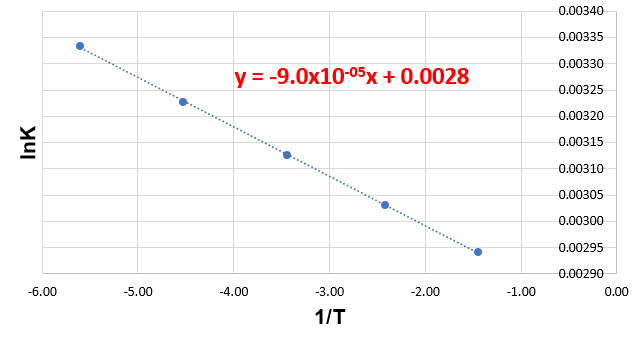# Problem: The following data show the rate constant of a reaction measured at several different temperatures.                   Temperature(K)                              Rate Constant (1/s)                           300                                                 3.37×10-3                           310                                                 1.08×10-2                           320                                                 3.21×10-2                           330                                                 8.96×10-2                           340                                                 0.235a. Use an Arrhenius plot to determine the activation barrier for the reaction.b. Use an Arrhenius plot to determine frequency factor for the reaction.

###### FREE Expert Solution

We have plotted the given data in lnk (y) vs. 1/T (x). The graph and line formula is:This means we need to use the two-point form of the Arrhenius Equation:

where:

m = slope

b = y-intercept

99% (70 ratings)###### Problem Details

The following data show the rate constant of a reaction measured at several different temperatures.
Temperature(K)                              Rate Constant (1/s)
300                                                 3.37×10-3
310                                                 1.08×10-2
320                                                 3.21×10-2
330                                                 8.96×10-2
340                                                 0.235

a. Use an Arrhenius plot to determine the activation barrier for the reaction.

b. Use an Arrhenius plot to determine frequency factor for the reaction.